# Calculating an Impact Parameter

In order to determine the amount of NSs required to account for the current amount of binary systems with at least one pulsar being spun up and one GS [GS + NS], we must calculate the impact parameter.This value is the smallest vertical distance a NS can be from the GS in the center which will still lead to its capture. As shown in the diagram below, we will call this value b.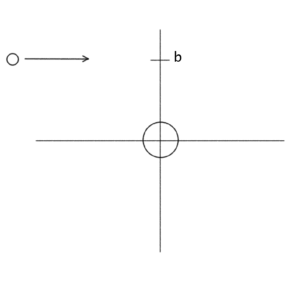In order to calculate the impact parameter we must account for the energy dissipated in tidal capture and the forces between the two bodies. We have,where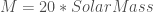is the mass of the GS,is the mass of the NS,is velocity of the NS andis the distance of closest approach which, for tidal capture is a minimum of 3*Radius of the GS which we will take to be roughly 100 solar radii.
Because our variables are astronomical, the addition of the 1 within the square root is negligible. Then, we have,and plugging in our values, we obtain an impact parameter of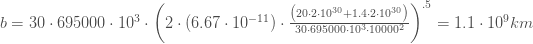Estimate of NSs required to produce the observations
Let us assume the Milky Way Galaxy is roughly 50 Kilo-Parsecs (kpc) in diameter or, since,
is about 162.5 light years (ly) in diameter and roughly 0.5 kpc thick. Then the volume would be,If we have 200 billion stars evenly dispersed then we can calculate: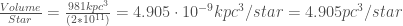or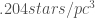which would place each star within a cube with side length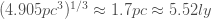and gives us an interstellar distance of the same, 5.52 ly. The closest star to the sun, Proxima Centauri is roughly 4 ly away.
So if we place each star within a 5.5 ly x 5.5 ly x 5.5 ly cube, the crosssectional area of the impact parameter would be given by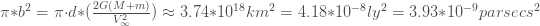If the neutron star is traveling 10 km/s and the length of the cube is 5 ly or 4.73*10^13 km, then it takes roughly 150,000 years to traverse the cube’s length.meaning we’d need 272 Giant Stars to 1 Neutron Star to create 1 binary every 150,000 years. Considering we know of roughly 100 such binaries with a lifespan of around 10 million years we’d need to produce 1 binary every 100,000 years and would thus needAlternatively, if we calculate the hit rate per 150000 years to produce 100 binaries in ten million years, we see thator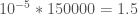an average of 1.5 hits per 150000. Thus we need a “hit rate” which istimes our current “hit rate.”

The easiest way to obtain this is to assume that there is not one neutron star per giant star but 181 giant stars per neutron star.

If we use a a “hit rate” of 1 in 10 billion and traversal time of 100,000 years, we have:assuming there is one neutron star per regular star over the course of 100,000 years in the Milky Way. In ten million years this would produce:so in ten million years we’d have 2000 Tidal Captures and would thus need to reduce the number of neutron stars by 20 for a ratio of 20 regular stars:1 neutron star in order to obtain 100 captures in ten million years.